F Distribution and One-Way ANOVA

# The F Distribution and the F-Ratio

The distribution used for the hypothesis test is a new one. It is called the F distribution, named after Sir Ronald Fisher, an English statistician. The F statistic is a ratio (a fraction). There are two sets of degrees of freedom; one for the numerator and one for the denominator.

For example, if F follows an F distribution and the number of degrees of freedom for the numerator is four, and the number of degrees of freedom for the denominator is ten, then F ~ F4,10.

Note

The F distribution is derived from the Student’s t-distribution. The values of the F distribution are squares of the corresponding values of the t-distribution. One-Way ANOVA expands the t-test for comparing more than two groups. The scope of that derivation is beyond the level of this course. It is preferable to use ANOVA when there are more than two groups instead of performing pairwise t-tests because performing multiple tests introduces the likelihood of making a Type 1 error.

To calculate the F ratio, two estimates of the variance are made.

1. Variance between samples: An estimate of σ2 that is the variance of the sample means multiplied by n (when the sample sizes are the same.). If the samples are different sizes, the variance between samples is weighted to account for the different sample sizes. The variance is also called variation due to treatment or explained variation.
2. Variance within samples: An estimate of σ2 that is the average of the sample variances (also known as a pooled variance). When the sample sizes are different, the variance within samples is weighted. The variance is also called the variation due to error or unexplained variation.
• SSbetween = the sum of squares that represents the variation among the different samples
• SSwithin = the sum of squares that represents the variation within samples that is due to chance.

To find a “sum of squares” means to add together squared quantities that, in some cases, may be weighted. We used sum of squares to calculate the sample variance and the sample standard deviation in Descriptive Statistics.

MS means “mean square.” MSbetween is the variance between groups, and MSwithin is the variance within groups.

Calculation of Sum of Squares and Mean Square

• k = the number of different groups
• nj = the size of the jth group
• sj = the sum of the values in the jth group
• n = total number of all the values combined (total sample size: ∑nj)
• x = one value: ∑x = ∑sj
• Sum of squares of all values from every group combined: ∑x2
• Between group variability: SStotal = ∑x2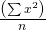• Total sum of squares: ∑x2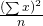• Explained variation: sum of squares representing variation among the different samples: SSbetween =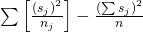• Unexplained variation: sum of squares representing variation within samples due to chance: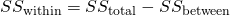• df‘s for different groups (df‘s for the numerator): df = k – 1
• Equation for errors within samples (df‘s for the denominator): dfwithin = nk
• Mean square (variance estimate) explained by the different groups: MSbetween =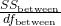• Mean square (variance estimate) that is due to chance (unexplained): MSwithin =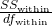MSbetween and MSwithin can be written as follows:

•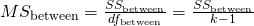•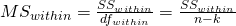The one-way ANOVA test depends on the fact that MSbetween can be influenced by population differences among means of the several groups. Since MSwithin compares values of each group to its own group mean, the fact that group means might be different does not affect MSwithin.

The null hypothesis says that all groups are samples from populations having the same normal distribution. The alternate hypothesis says that at least two of the sample groups come from populations with different normal distributions. If the null hypothesis is true, MSbetween and MSwithin should both estimate the same value.

Note

The null hypothesis says that all the group population means are equal. The hypothesis of equal means implies that the populations have the same normal distribution, because it is assumed that the populations are normal and that they have equal variances.

F-Ratio or F Statistic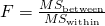If MSbetween and MSwithin estimate the same value (following the belief that H0 is true), then the F-ratio should be approximately equal to one. Mostly, just sampling errors would contribute to variations away from one. As it turns out, MSbetween consists of the population variance plus a variance produced from the differences between the samples. MSwithin is an estimate of the population variance. Since variances are always positive, if the null hypothesis is false, MSbetween will generally be larger than MSwithin.Then the F-ratio will be larger than one. However, if the population effect is small, it is not unlikely that MSwithin will be larger in a given sample.

The foregoing calculations were done with groups of different sizes. If the groups are the same size, the calculations simplify somewhat and the F-ratio can be written as:

F-Ratio Formula when the groups are the same size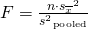where …
• n = the sample size
• dfnumerator = k – 1
• dfdenominator = nk
• s2 pooled = the mean of the sample variances (pooled variance)
•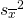= the variance of the sample means

Data are typically put into a table for easy viewing. One-Way ANOVA results are often displayed in this manner by computer software.

Source of Variation Sum of Squares (SS) Degrees of Freedom (df) Mean Square (MS) F
Factor
(Between)
SS(Factor) k – 1 MS(Factor) = SS(Factor)/(k – 1) F = MS(Factor)/MS(Error)
Error
(Within)
SS(Error) nk MS(Error) = SS(Error)/(nk)
Total SS(Total) n – 1

Three different diet plans are to be tested for mean weight loss. The entries in the table are the weight losses for the different plans. The one-way ANOVA results are shown in (Figure).

Plan 1: n1 = 4 Plan 2: n2 = 3 Plan 3: n3 = 3
5 3.5 8
4.5 7 4
4 3.5
3 4.5

s1 = 16.5, s2 =15, s3 = 15.5

Following are the calculations needed to fill in the one-way ANOVA table. The table is used to conduct a hypothesis test.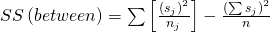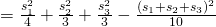where n1 = 4, n2 = 3, n3 = 3 and n = n1 + n2 + n3 = 10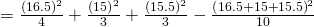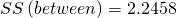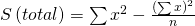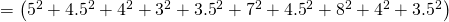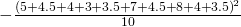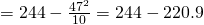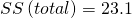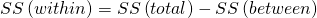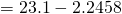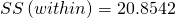One-Way ANOVA Table: The formulas for SS(Total), SS(Factor) = SS(Between) and SS(Error) = SS(Within) as shown previously. The same information is provided by the TI calculator hypothesis test function ANOVA in STAT TESTS (syntax is ANOVA(L1, L2, L3) where L1, L2, L3 have the data from Plan 1, Plan 2, Plan 3 respectively).

Source of Variation Sum of Squares (SS) Degrees of Freedom (df) Mean Square (MS) F
Factor
(Between)
SS(Factor)
= SS(Between)
= 2.2458
k – 1
= 3 groups – 1
= 2
MS(Factor)
= SS(Factor)/(k – 1)
= 2.2458/2
= 1.1229
F =
MS(Factor)/MS(Error)
= 1.1229/2.9792
= 0.3769
Error
(Within)
SS(Error)
= SS(Within)
= 20.8542
nk
= 10 total data – 3 groups
= 7
MS(Error)
= SS(Error)/(nk)
= 20.8542/7
= 2.9792
Total SS(Total)
= 2.2458 + 20.8542
= 23.1
n – 1
= 10 total data – 1
= 9
Try It

As part of an experiment to see how different types of soil cover would affect slicing tomato production, Marist College students grew tomato plants under different soil cover conditions. Groups of three plants each had one of the following treatments

• bare soil
• a commercial ground cover
• black plastic
• straw
• compost

All plants grew under the same conditions and were the same variety. Students recorded the weight (in grams) of tomatoes produced by each of the n = 15 plants:

Bare: n1 = 3 Ground Cover: n2 = 3 Plastic: n3 = 3 Straw: n4 = 3 Compost: n5 = 3
2,625 5,348 6,583 7,285 6,277
2,997 5,682 8,560 6,897 7,818
4,915 5,482 3,830 9,230 8,677

Create the one-way ANOVA table.

The one-way ANOVA hypothesis test is always right-tailed because larger F-values are way out in the right tail of the F-distribution curve and tend to make us reject H0.

### Notation

The notation for the F distribution is F ~ Fdf(num),df(denom)

where df(num) = dfbetween and df(denom) = dfwithin

The mean for the F distribution is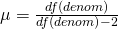### References

Tomato Data, Marist College School of Science (unpublished student research)

### Chapter Review

Analysis of variance compares the means of a response variable for several groups. ANOVA compares the variation within each group to the variation of the mean of each group. The ratio of these two is the F statistic from an F distribution with (number of groups – 1) as the numerator degrees of freedom and (number of observations – number of groups) as the denominator degrees of freedom. These statistics are summarized in the ANOVA table.

### Formula Review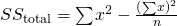dfbetween = df(num) = k – 1

dfwithin = df(denom) = nk

MSbetween =MSwithin =F =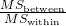F ratio when the groups are the same size: F =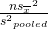Mean of the F distribution: µ =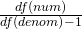where:

• k = the number of groups
• nj = the size of the jth group
• sj = the sum of the values in the jth group
• n = the total number of all values (observations) combined
• x = one value (one observation) from the data
•= the variance of the sample means
•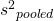= the mean of the sample variances (pooled variance)

Use the following information to answer the next eight exercises. Groups of men from three different areas of the country are to be tested for mean weight. The entries in (Figure) are the weights for the different groups.

Group 1 Group 2 Group 3
216 202 170
198 213 165
240 284 182
187 228 197
176 210 201

What is the Sum of Squares Factor?

4,939.2

What is the Sum of Squares Error?

What is the df for the numerator?

2

What is the df for the denominator?

What is the Mean Square Factor?

2,469.6

What is the Mean Square Error?

What is the F statistic?

3.7416

Use the following information to answer the next eight exercises. Girls from four different soccer teams are to be tested for mean goals scored per game. The entries in the table are the goals per game for the different teams. The one-way ANOVA results are shown in (Figure).

Team 1 Team 2 Team 3 Team 4
1 2 0 3
2 3 1 4
0 2 1 4
3 4 0 3
2 4 0 2

What is SSbetween?

What is the df for the numerator?

3

What is MSbetween?

What is SSwithin?

13.2

What is the df for the denominator?

What is MSwithin?

0.825

What is the F statistic?

Judging by the F statistic, do you think it is likely or unlikely that you will reject the null hypothesis?

Because a one-way ANOVA test is always right-tailed, a high F statistic corresponds to a low p-value, so it is likely that we will reject the null hypothesis.

### Homework

Use the following information to answer the next three exercises. Suppose a group is interested in determining whether teenagers obtain their drivers licenses at approximately the same average age across the country. Suppose that the following data are randomly collected from five teenagers in each region of the country. The numbers represent the age at which teenagers obtained their drivers licenses.

Northeast South West Central East
16.3 16.9 16.4 16.2 17.1
16.1 16.5 16.5 16.6 17.2
16.4 16.4 16.6 16.5 16.6
16.5 16.2 16.1 16.4 16.8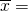________ ________ ________ ________ ________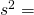________ ________ ________ ________ ________

H0: µ1 = µ2 = µ3 = µ4 = µ5

: At least any two of the group means µ1, µ2, …, µ5 are not equal.

degrees of freedom – numerator: df(num) = _________

degrees of freedom – denominator: df(denom) = ________

df(denom) = 15

F statistic = ________